# Using QUERY and understanding the Query Language

Filter and transform data from a range of cells using an SQL-like language.

### What is the QUERY function

The QUERY function in Rows allows you to use SQL (Structured Query Language) to analyze and manipulate data in your spreadsheet. It is a very powerful and versatile function that can be used to perform a wide variety of tasks, such as filtering data, calculating statistics, and even joining data from multiple sheets or tables.

### How does the QUERY function work

The syntax for the QUERY function looks like this; =QUERY(data,query,[headers])

#### It takes 3 arguments

• data
• query

Let's first define what these 3 mean.

#### data

This is the data from the spreadsheet range you want to query. There are 4 ways the data can be expressed;

• As something distinct, such as A1
• As an entire column, such as A
• As literals, such as 'Jack & Jill' NOTE: Each literal is separated by a comma and would be placed in its own column.
• As an aggregate function, such as SUM, COUNT, AVERAGE, MIN & MAX. Note: Aggregate functions are mostly used with the GROUP BY clause.

#### query

This is the SQL command that will be used against the data provided. The SQL command consists of several clauses, some of which are mandatory and others which are optional. Below is a definitive list of all clauses available within Rows.

Leaving this argument empty will result in a "SELECT *" command that will return all results from the requested data.

This is how many rows are considered the headers of the data. The default is 1.

### Query commands

Below we will outline the basics of what can be done through SQL, but we highly advise familiarising yourself with SQL through other educational content.

It is important to note that the clauses should always be used in this order;

1. select
2. where
3. group by
4. pivot
5. order by
6. limit
7. label

#### SELECT

This supports 2 methods;

Selections with SELECT or mathematical operations with SUM, MIN, MAX, AVERAGE, COUNT.

#### WHERE

WHERE is a conditional clause used to filter the data. It accepts all arithmetic operators, such as;

• = is equal to
• != is not equal to
• > is greater than
• < is less than
• => is greater than or equal to
• =< is less than or equal to
• contains
• like
• is not null
• is null
• starts with
• ends with
• matches: matches uses regular expressions to evaluate the match between the value and the syntax in the where clause:
• Use '' to for exact matches (e.g. `WHERE B MATCHES 'Bananas'`)
• Use . for partial matches (e.g. `WHERE B MATCHES '.*ana.'`)

Note: Strings will need to be wrapped in single quotes but numbers should not be.

#### Group By

GROUP BY allows you to group the result set by one or more columns, providing a more granular view of the data.

It can be useful when you want to summarize or analyze data in your spreadsheet by groups or categories. For example, you could use the GROUP BY clause to calculate the average cost, maximum quantity, or total sales for each product category.

It is typically used in combination with one or more aggregate functions, such as SUM, AVG, MIN, MAX, or COUNT, to perform calculations on the grouped data.

For example, consider the table below;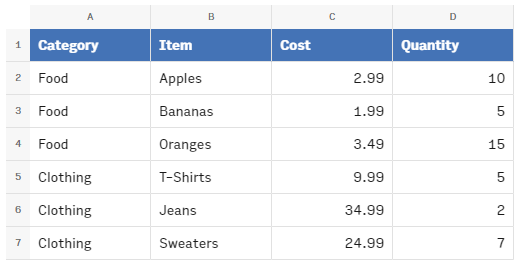If we wanted to create a pivot table that shows the total cost of each item within each category, we can use the following QUERY function:

`=QUERY(A1:D7,"SELECT A, SUM(C) GROUP BY A")`

This produces the following table: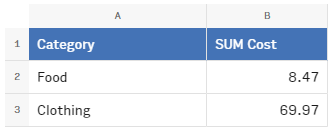#### Pivot

PIVOT is used to rearrange and summarize the data in a table. It allows you to transpose the data from a horizontal orientation (rows) to a vertical orientation (columns), or vice versa. This can be useful for creating pivot tables, which are a common way of summarizing and analyzing large sets of data.

Take the table below as an example:For example, if we wanted to create a pivot table that shows the total cost of each item within each category, we could use the following QUERY function:

`=QUERY(A2:D7,"SELECT A, SUM(C) group by A PIVOT B")`

In this example, the PIVOT clause is used to convert the Item data from a horizontal orientation (rows) to a vertical orientation (columns), with one column for each Item. The SUM function is used to calculate the total quantity sold for each product. The result of this would be the table below: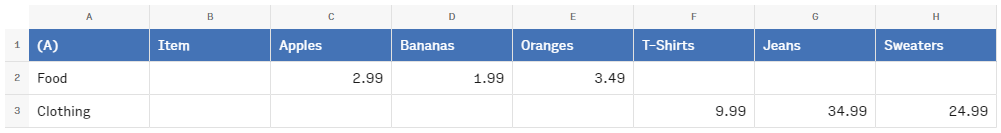#### Order By

ORDER BY is used in the QUERY function to sort the rows of a table based on the values in one or more columns.

It can be useful when you want to present your query results in a specific order, e.g.alphabetical, by date, or by a custom order that you specify.

Ascending order is the default. You can also use the DESC keyword with the ORDER BY clause to specify that the rows should be sorted in descending order.e.g. to sort in reverse alphabetical order, by the latest date, or by the highest or lowest values in a column.For example, if we wanted to create a table that shows the cost of each item within each category, sorted by the cost in descending order, we could use the following QUERY function:

`=QUERY(A1:D7,"SELECT A, B, SUM(C) GROUP BY A, B ORDER BY sum(C) DESC")`

This would produce the following table: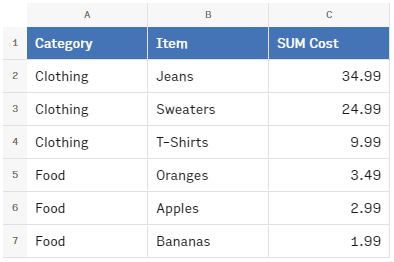#### Limit

LIMIT is used in the QUERY function to specify the maximum number of rows that a query should return.

It can be useful when you have a large dataset and only want to display a limited number of rows in your query results. For example, you could use the LIMIT clause to display the top 10 most expensive items, the top 5 best-selling products, or the first 100 entries in a database.For example, if we wanted to create a table that shows the cost of the most expensive three items, we could use the following QUERY function:

`=QUERY(A1:D7,"SELECT A, B, SUM(C) GROUP BY A,B ORDER BY SUM(C) DESC LIMIT 3")`

This would produce the following table: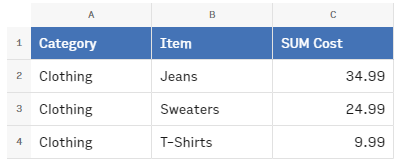#### Offset

OFFSET is used in the QUERY function to specify the number of rows to skip at the beginning of the table before returning the query results.

It can be useful when you want to paginate your query results or display only a portion of the data in your table. For example, you could use the OFFSET clause to display the next 10 items in a list, the previous 5 entries in a database, or the second half of a large dataset.

You can also use the LIMIT clause in combination with the OFFSET clause to specify the number of rows to return in your query results.For example, if we wanted to create a table that shows the cost of the first three items within each category, starting from the second item, we could use the following QUERY function:

`=QUERY(A1:D7,"SELECT A, B, SUM(C) GROUP BY A,B ORDER BY SUM(C) DESC OFFSET 1")`

This would produce the following table: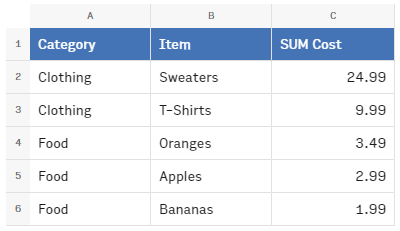#### Label

Label is used to rename the headers of the columns in the QUERY function. Use the following syntax to rename a colum:

``1label column_id label_string``

Example:

`=QUERY(A1:D7,"SELECT * Label A 'Cat', B 'Type'")`

This will label column A with Cat and col B with Type.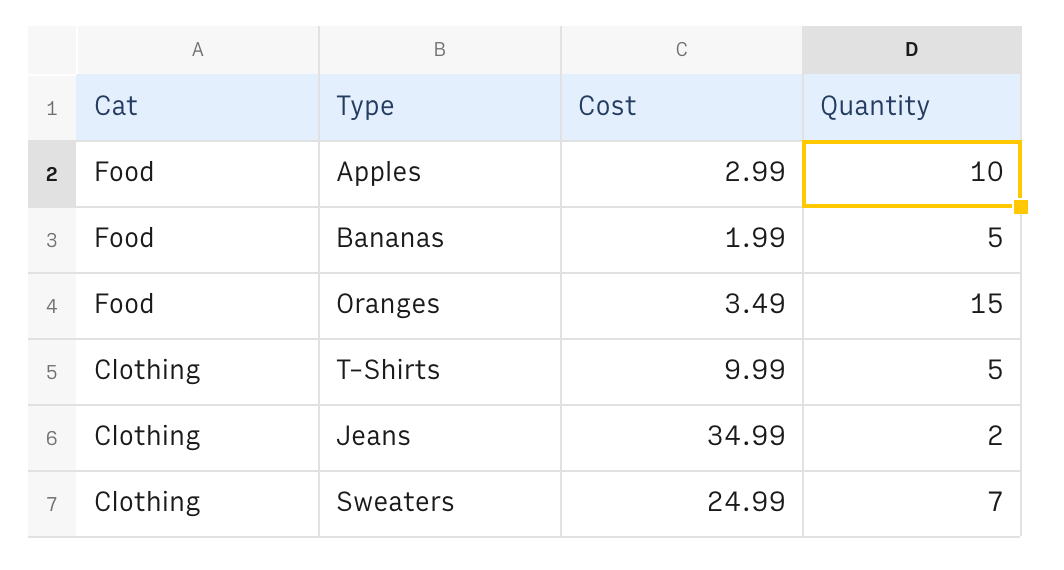### How Rows is different from Google Sheets’ QUERY() function

The third argument (headers argument) functions a little differently in both languages.

• If the argument is not specified, Google tries to identify the header row(s) automatically from the range argument, it can match one or more rows;
• If the argument is specified then it will respect the nr of header rows;
• Rows
• If the argument is not specified, no header rows are identified; Same as if the argument value=0;
• If the argument is specified then it will respect the number of header rows; There is an exception to this rule in Rows which is when you use the pivot clause. This clause will automatically produce headers based on the columns it specifies.

### Current limitations and planned improvements

• Scalar Functions
• Select time(B), date(B), upper(A), lower(A)
• Arithmetic Operators
• Select 2 * A, -1 + B, sum(C) / 2
• Update/Set/Insert/Delete
• Set A=2, B=C, D='updated' Where F>2
• Rows formatter/editor
• Clause Options
• Where A='Some Text' Options CASE_INSENSITIVE
• Improve date/time handling
• Select Where A='2022-10-02' Select Where date(A)='2022-10-02' Select * Where A=date('2022-10-02' )

### Common mistakes to avoid with QUERY()

Here are some common mistakes that people make when using the QUERY function in Rows:

• Forgetting to enclose the query in quotes: The query parameter of the QUERY function must be enclosed in quotation marks, otherwise it will not be recognized as a string and will produce an error.
• Using the wrong column or cell references: It is important to use the correct column and cell references in your query, otherwise you may get incorrect or unexpected results.
• Using the wrong syntax: SQL has a specific syntax that must be followed in order for the query to be valid. If you make a mistake with the syntax, your query will not work as expected.

### Tips and tricks

• Wrap your QUERY formula in the expand formula to automatically expand the resulting JSON from the query into a table.
• From: `=QUERY(A1:D7,"SELECT A, B, SUM(C) GROUP BY A,B ORDER BY SUM(C) DESC OFFSET 1")`
• To: `=EXPAND(QUERY(A1:D7,"SELECT A, B, SUM(C) GROUP BY A,B ORDER BY SUM(C) DESC OFFSET 1"))`